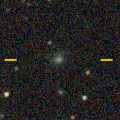# \$B%Q%m%^!

P/2019 LM4 ( Palomar )###\$B%W%m%U%#!<%k(B

 \$BH/8+F|(B 2019\$BG/(B6\$B7n(B4\$BF|(B \$BH/8+8wEY(B 19.0\$BEy(B \$BH/8+ Q.-Z. Ye (Palomar Mountain), Z. T. F. Collaboration

###\$B###\$B50F;MWAG(B

```Epoch 2019 June 6.0 TT = JDT 2458640.5
T 2019 June 18.22448 TT                                 Rudenko
q   2.3713239            (2000.0)            P               Q
n   0.07205302     Peri.   68.35861     -0.60626915     -0.55763133
a   5.7196299      Node    72.85839     +0.30627189     -0.82169601
e   0.5854061      Incl.   36.39536     +0.73391774     -0.11774110
P  13.7
From 224 observations 2019 June 4-2020 June 16, mean residual 0".5.
```

###\$B8wEYJQ2=(B

```        m1 =  12.5 + 5 log\$B&\$(B +  10.0 log r           [   ,328]  (              \$B!A(B2020\$BG/(B 5\$B7n(B11\$BF|(B)
m1 = -46   + 5 log\$B&\$(B + 100   log r(t + 100)  [328,400]  (2020\$BG/(B 5\$B7n(B11\$BF|!A(B2020\$BG/(B 7\$B7n(B22\$BF|(B)
m1 =  12.5 + 5 log\$B&\$(B +  10.0 log r           [400,   ]  (2020\$BG/(B 7\$B7n(B22\$BF|!A(B              )
```##### \$B50F;MWAG\$O(BM.P.E.C. 2020-P19\$B\$K7G:\\$5\$l\$?\$b\$N\$G\$9!#(B \$B8wEY%0%i%U\$O(BComet for Windows\$B\$G:n@.\$7\$?\$b\$N\$G\$9!#(B